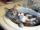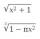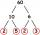# Integers + multiplication - examples

1. CatsTwo cats caught two mice in two days. How many mouses will catch 6 cats for 6 days?
2. Unknown xIf we add to unknown number 21, then divide by 6 and then subtract 51, we get back an unknown number. What is this unknown number?
3. NumberCalculate the integer number which, divided by 34 gives 10 and the rest 25.
4. With bracketCalculate (evaluate) simple mathematical expression with a negative numbers and a bracket: 13+15*5-2*(-6)
5. ValueDetermine the value of the following exspressions: a) (23-25)·(4-5) b) (97-123):(18+8)
6. Expression 6Evaluate expression: -6-2(4-8)-9
7. Brackets 2Add parenthesis to make true: 5-2×6-4+2=5
8. Progression12, 60, -300,1500 need next 2 numbers of pattern
9. Expression plus minusEvaluate expression: (-1)2 . 12 – 6 : 3 + (-3) . (-2) + 22 – (-3) . 2
10. SimplifySimplify expression - which expression is equivalent to: 3(m + 2) − 4(2m − 9)
11. DozenWhat is the product of 26 and 5? Write the answer in Arabic numeral. Add up the digits. How many of this is in a dozen? Divide #114 by this

We apologize, but in this category are not a lot of examples.
Do you have an interesting mathematical example that you can't solve it? Enter it, and we can try to solve it.

To this e-mail address, we will reply solution; solved examples are also published here. Please enter e-mail correctly and check whether you don't have a full mailbox.